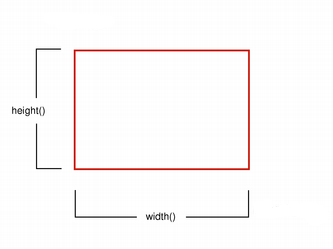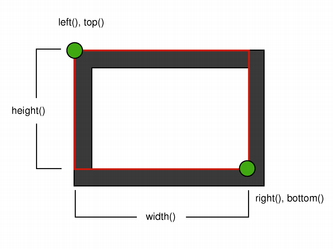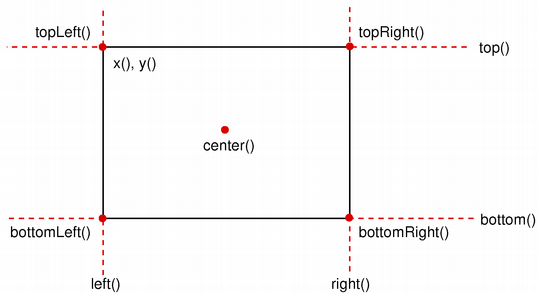#### Previous topic

QTextBoundaryFinder

QRect

# QRectF¶

## Detailed Description¶

The PySide.QtCore.QRectF class defines a rectangle in the plane using floating point precision.

A rectangle is normally expressed as an upper-left corner and a size. The size (width and height) of a PySide.QtCore.QRectF is always equivalent to the mathematical rectangle that forms the basis for its rendering.

A PySide.QtCore.QRectF can be constructed with a set of left, top, width and height integers, or from a PySide.QtCore.QPoint and a PySide.QtCore.QSize . The following code creates two identical rectangles.

```r1 = QRectF(100, 200, 11, 16)
r2 = QRectF(QPoint(100, 200), QSize(11, 16))
```

There is also a third constructor creating a PySide.QtCore.QRectF from a PySide.QtCore.QRect , and a corresponding PySide.QtCore.QRectF.toRect() function that returns a PySide.QtCore.QRect object based on the values of this rectangle (note that the coordinates in the returned rectangle are rounded to the nearest integer).

The PySide.QtCore.QRectF class provides a collection of functions that return the various rectangle coordinates, and enable manipulation of these. PySide.QtCore.QRectF also provide functions to move the rectangle relative to the various coordinates. In addition there is a PySide.QtCore.QRectF.moveTo() function that moves the rectangle, leaving its top left corner at the given coordinates. Alternatively, the PySide.QtCore.QRectF.translate() function moves the rectangle the given offset relative to the current position, and the PySide.QtCore.QRectF.translated() function returns a translated copy of this rectangle.

The PySide.QtCore.QRectF.size() function returns the rectange’s dimensions as a PySide.QtCore.QSize . The dimensions can also be retrieved separately using the PySide.QtCore.QRectF.width() and PySide.QtCore.QRectF.height() functions. To manipulate the dimensions use the PySide.QtCore.QRectF.setSize() , PySide.QtCore.QRectF.setWidth() or PySide.QtCore.QRectF.setHeight() functions. Alternatively, the size can be changed by applying either of the functions setting the rectangle coordinates, for example, PySide.QtCore.QRectF.setBottom() or PySide.QtCore.QRectF.setRight() .

The PySide.QtCore.QRectF.contains() function tells whether a given point is inside the rectangle or not, and the PySide.QtCore.QRectF.intersects() function returns true if this rectangle intersects with a given rectangle (otherwise false). The PySide.QtCore.QRectF class also provides the PySide.QtCore.QRectF.intersected() function which returns the intersection rectangle, and the PySide.QtCore.QRectF.united() function which returns the rectangle that encloses the given rectangle and this:PySide.QtCore.QRectF.intersected() PySide.QtCore.QRectF.united()

The PySide.QtCore.QRectF.isEmpty() function returns true if the rectangle’s width or height is less than, or equal to, 0. Note that an empty rectangle is not valid: The PySide.QtCore.QRectF.isValid() function returns true if both width and height is larger than 0. A null rectangle ( PySide.QtCore.QRectF.isNull() == true) on the other hand, has both width and height set to 0.

Note that due to the way PySide.QtCore.QRect and PySide.QtCore.QRectF are defined, an empty PySide.QtCore.QRectF is defined in essentially the same way as PySide.QtCore.QRect .

Finally, PySide.QtCore.QRectF objects can be streamed as well as compared.

### Rendering¶

When using an anti-aliased painter, the boundary line of a PySide.QtCore.QRectF will be rendered symmetrically on both sides of the mathematical rectangle’s boundary line. But when using an aliased painter (the default) other rules apply.

Then, when rendering with a one pixel wide pen the PySide.QtCore.QRectF ‘s boundary line will be rendered to the right and below the mathematical rectangle’s boundary line.

When rendering with a two pixels wide pen the boundary line will be split in the middle by the mathematical rectangle. This will be the case whenever the pen is set to an even number of pixels, while rendering with a pen with an odd number of pixels, the spare pixel will be rendered to the right and below the mathematical rectangle as in the one pixel case.Logical representation One pixel wide penTwo pixel wide pen Three pixel wide pen

### Coordinates¶

The PySide.QtCore.QRectF class provides a collection of functions that return the various rectangle coordinates, and enable manipulation of these. PySide.QtCore.QRectF also provide functions to move the rectangle relative to the various coordinates.

For example: the PySide.QtCore.QRectF.bottom() , PySide.QtCore.QRectF.setBottom() and PySide.QtCore.QRectF.moveBottom() functions: PySide.QtCore.QRectF.bottom() returns the y-coordinate of the rectangle’s bottom edge, PySide.QtCore.QRectF.setBottom() sets the bottom edge of the rectangle to the given y coordinate (it may change the height, but will never change the rectangle’s top edge) and PySide.QtCore.QRectF.moveBottom() moves the entire rectangle vertically, leaving the rectangle’s bottom edge at the given y coordinate and its size unchanged.It is also possible to add offsets to this rectangle’s coordinates using the PySide.QtCore.QRectF.adjust() function, as well as retrieve a new rectangle based on adjustments of the original one using the PySide.QtCore.QRectF.adjusted() function. If either of the width and height is negative, use the PySide.QtCore.QRectF.normalized() function to retrieve a rectangle where the corners are swapped.

In addition, PySide.QtCore.QRectF provides the PySide.QtCore.QRectF.getCoords() function which extracts the position of the rectangle’s top-left and bottom-right corner, and the PySide.QtCore.QRectF.getRect() function which extracts the rectangle’s top-left corner, width and height. Use the PySide.QtCore.QRectF.setCoords() and PySide.QtCore.QRectF.setRect() function to manipulate the rectangle’s coordinates and dimensions in one go.

class PySide.QtCore.QRectF
class PySide.QtCore.QRectF(topleft, bottomRight)
class PySide.QtCore.QRectF(topleft, size)
class PySide.QtCore.QRectF(rect)
class PySide.QtCore.QRectF(QRectF)
class PySide.QtCore.QRectF(left, top, width, height)
Parameters: width – PySide.QtCore.qreal size – PySide.QtCore.QSizeF bottomRight – PySide.QtCore.QPointF rect – PySide.QtCore.QRect QRectF – PySide.QtCore.QRectF topleft – PySide.QtCore.QPointF left – PySide.QtCore.qreal height – PySide.QtCore.qreal top – PySide.QtCore.qreal

Constructs a null rectangle.

Constructs a rectangle with the given topLeft and bottomRight corners.

Constructs a rectangle with the given topLeft corner and the given size .

Constructs a PySide.QtCore.QRectF rectangle from the given PySide.QtCore.QRect rectangle .

Constructs a rectangle with (x , y ) as its top-left corner and the given width and height .

PySide.QtCore.QRectF.__reduce__()
Return type: PyObject
PySide.QtCore.QRectF.__repr__()
Return type: PyObject
Parameters: x1 – PySide.QtCore.qreal y1 – PySide.QtCore.qreal x2 – PySide.QtCore.qreal y2 – PySide.QtCore.qreal

Adds dx1 , dy1 , dx2 and dy2 respectively to the existing coordinates of the rectangle.

Parameters: x1 – PySide.QtCore.qreal y1 – PySide.QtCore.qreal x2 – PySide.QtCore.qreal y2 – PySide.QtCore.qreal PySide.QtCore.QRectF

Returns a new rectangle with dx1 , dy1 , dx2 and dy2 added respectively to the existing coordinates of this rectangle.

PySide.QtCore.QRectF.bottom()
Return type: PySide.QtCore.qreal

Returns the y-coordinate of the rectangle’s bottom edge.

PySide.QtCore.QRectF.bottomLeft()
Return type: PySide.QtCore.QPointF

Returns the position of the rectangle’s bottom-left corner.

PySide.QtCore.QRectF.bottomRight()
Return type: PySide.QtCore.QPointF

Returns the position of the rectangle’s bottom-right corner.

PySide.QtCore.QRectF.center()
Return type: PySide.QtCore.QPointF

Returns the center point of the rectangle.

PySide.QtCore.QRectF.contains(x, y)
Parameters: x – PySide.QtCore.qreal y – PySide.QtCore.qreal PySide.QtCore.bool

Returns true if the point (x , y ) is inside or on the edge of the rectangle; otherwise returns false.

PySide.QtCore.QRectF.contains(p)
Parameters: p – PySide.QtCore.QPointF PySide.QtCore.bool

Returns true if the given point is inside or on the edge of the rectangle; otherwise returns false.

PySide.QtCore.QRectF.contains(r)
Parameters: r – PySide.QtCore.QRectF PySide.QtCore.bool

Returns true if the given rectangle is inside this rectangle; otherwise returns false.

PySide.QtCore.QRectF.getCoords()

Extracts the position of the rectangle’s top-left corner to *``x1`` and *``y1`` , and the position of the bottom-right corner to *``x2`` and *``y2`` .

PySide.QtCore.QRectF.getRect()

Extracts the position of the rectangle’s top-left corner to *``x`` and *``y`` , and its dimensions to *``width`` and *``height`` .

PySide.QtCore.QRectF.height()
Return type: PySide.QtCore.qreal

Returns the height of the rectangle.

PySide.QtCore.QRectF.intersect(r)
Parameters: r – PySide.QtCore.QRectF PySide.QtCore.QRectF

PySide.QtCore.QRectF.intersected(other)
Parameters: other – PySide.QtCore.QRectF PySide.QtCore.QRectF

Returns the intersection of this rectangle and the given rectangle . Note that r.intersected(s) is equivalent to r & s .PySide.QtCore.QRectF.intersects() PySide.QtCore.QRectF.united() PySide.QtCore.QRectF.operator&=()

PySide.QtCore.QRectF.intersects(r)
Parameters: r – PySide.QtCore.QRectF PySide.QtCore.bool

Returns true if this rectangle intersects with the given rectangle (i.e. there is a non-empty area of overlap between them), otherwise returns false.

The intersection rectangle can be retrieved using the PySide.QtCore.QRectF.intersected() function.

PySide.QtCore.QRectF.isEmpty()
Return type: PySide.QtCore.bool

Returns true if the rectangle is empty, otherwise returns false.

An empty rectangle has PySide.QtCore.QRectF.width() <= 0 or PySide.QtCore.QRectF.height() <= 0. An empty rectangle is not valid (i.e., PySide.QtCore.QRectF.isEmpty() == ! PySide.QtCore.QRectF.isValid() ).

Use the PySide.QtCore.QRectF.normalized() function to retrieve a rectangle where the corners are swapped.

PySide.QtCore.QRectF.isNull()
Return type: PySide.QtCore.bool

Returns true if the rectangle is a null rectangle, otherwise returns false.

A null rectangle has both the width and the height set to 0. A null rectangle is also empty, and hence not valid.

PySide.QtCore.QRectF.isValid()
Return type: PySide.QtCore.bool

Returns true if the rectangle is valid, otherwise returns false.

A valid rectangle has a PySide.QtCore.QRectF.width() > 0 and PySide.QtCore.QRectF.height() > 0. Note that non-trivial operations like intersections are not defined for invalid rectangles. A valid rectangle is not empty (i.e., PySide.QtCore.QRectF.isValid() == ! PySide.QtCore.QRectF.isEmpty() ).

PySide.QtCore.QRectF.left()
Return type: PySide.QtCore.qreal

Returns the x-coordinate of the rectangle’s left edge. Equivalent to PySide.QtCore.QRectF.x() .

PySide.QtCore.QRectF.moveBottom(pos)
Parameters: pos – PySide.QtCore.qreal

Moves the rectangle vertically, leaving the rectangle’s bottom edge at the given y coordinate. The rectangle’s size is unchanged.

PySide.QtCore.QRectF.moveBottomLeft(p)
Parameters: p – PySide.QtCore.QPointF

Moves the rectangle, leaving the bottom-left corner at the given position . The rectangle’s size is unchanged.

PySide.QtCore.QRectF.moveBottomRight(p)
Parameters: p – PySide.QtCore.QPointF

Moves the rectangle, leaving the bottom-right corner at the given position . The rectangle’s size is unchanged.

PySide.QtCore.QRectF.moveCenter(p)
Parameters: p – PySide.QtCore.QPointF

Moves the rectangle, leaving the center point at the given position . The rectangle’s size is unchanged.

PySide.QtCore.QRectF.moveLeft(pos)
Parameters: pos – PySide.QtCore.qreal

Moves the rectangle horizontally, leaving the rectangle’s left edge at the given x coordinate. The rectangle’s size is unchanged.

PySide.QtCore.QRectF.moveRight(pos)
Parameters: pos – PySide.QtCore.qreal

Moves the rectangle horizontally, leaving the rectangle’s right edge at the given x coordinate. The rectangle’s size is unchanged.

PySide.QtCore.QRectF.moveTo(x, t)
Parameters: x – PySide.QtCore.qreal t – PySide.QtCore.qreal

Moves the rectangle, leaving the top-left corner at the given position (x , y ). The rectangle’s size is unchanged.

PySide.QtCore.QRectF.moveTo(p)
Parameters: p – PySide.QtCore.QPointF

Moves the rectangle, leaving the top-left corner at the given position .

PySide.QtCore.QRectF.moveTop(pos)
Parameters: pos – PySide.QtCore.qreal

Moves the rectangle vertically, leaving the rectangle’s top line at the given y coordinate. The rectangle’s size is unchanged.

PySide.QtCore.QRectF.moveTopLeft(p)
Parameters: p – PySide.QtCore.QPointF

Moves the rectangle, leaving the top-left corner at the given position . The rectangle’s size is unchanged.

PySide.QtCore.QRectF.moveTopRight(p)
Parameters: p – PySide.QtCore.QPointF

Moves the rectangle, leaving the top-right corner at the given position . The rectangle’s size is unchanged.

PySide.QtCore.QRectF.normalized()
Return type: PySide.QtCore.QRectF

Returns a normalized rectangle; i.e., a rectangle that has a non-negative width and height.

If PySide.QtCore.QRectF.width() < 0 the function swaps the left and right corners, and it swaps the top and bottom corners if PySide.QtCore.QRectF.height() < 0.

PySide.QtCore.QRectF.__ne__(arg__2)
Parameters: arg__2 – PySide.QtCore.QRectF PySide.QtCore.bool
PySide.QtCore.QRectF.__and__(r)
Parameters: r – PySide.QtCore.QRectF PySide.QtCore.QRectF

Returns the intersection of this rectangle and the given rectangle . Returns an empty rectangle if there is no intersection.

PySide.QtCore.QRectF.operator&=() PySide.QtCore.QRectF.intersected()

PySide.QtCore.QRectF.__iand__(r)
Parameters: r – PySide.QtCore.QRectF PySide.QtCore.QRectF

Intersects this rectangle with the given rectangle .

PySide.QtCore.QRectF.intersected() PySide.QtCore.QRectF.operator|=()

PySide.QtCore.QRectF.__eq__(arg__2)
Parameters: arg__2 – PySide.QtCore.QRectF PySide.QtCore.bool
PySide.QtCore.QRectF.__or__(r)
Parameters: r – PySide.QtCore.QRectF PySide.QtCore.QRectF

Returns the bounding rectangle of this rectangle and the given rectangle .

PySide.QtCore.QRectF.united() PySide.QtCore.QRectF.operator|=()

PySide.QtCore.QRectF.__ior__(r)
Parameters: r – PySide.QtCore.QRectF PySide.QtCore.QRectF

Unites this rectangle with the given rectangle .

PySide.QtCore.QRectF.united() PySide.QtCore.QRectF.operator|()

PySide.QtCore.QRectF.right()
Return type: PySide.QtCore.qreal

Returns the x-coordinate of the rectangle’s right edge.

PySide.QtCore.QRectF.setBottom(pos)
Parameters: pos – PySide.QtCore.qreal

Sets the bottom edge of the rectangle to the given y coordinate. May change the height, but will never change the top edge of the rectangle.

PySide.QtCore.QRectF.setBottomLeft(p)
Parameters: p – PySide.QtCore.QPointF

Set the bottom-left corner of the rectangle to the given position . May change the size, but will never change the top-right corner of the rectangle.

PySide.QtCore.QRectF.setBottomRight(p)
Parameters: p – PySide.QtCore.QPointF

Set the bottom-right corner of the rectangle to the given position . May change the size, but will never change the top-left corner of the rectangle.

PySide.QtCore.QRectF.setCoords(x1, y1, x2, y2)
Parameters: x1 – PySide.QtCore.qreal y1 – PySide.QtCore.qreal x2 – PySide.QtCore.qreal y2 – PySide.QtCore.qreal

Sets the coordinates of the rectangle’s top-left corner to (x1 , y1 ), and the coordinates of its bottom-right corner to (x2 , y2 ).

PySide.QtCore.QRectF.setHeight(h)
Parameters: h – PySide.QtCore.qreal

Sets the height of the rectangle to the given height . The bottom edge is changed, but not the top one.

PySide.QtCore.QRectF.setLeft(pos)
Parameters: pos – PySide.QtCore.qreal

Sets the left edge of the rectangle to the given x coordinate. May change the width, but will never change the right edge of the rectangle.

Equivalent to PySide.QtCore.QRectF.setX() .

PySide.QtCore.QRectF.setRect(x, y, w, h)
Parameters: x – PySide.QtCore.qreal y – PySide.QtCore.qreal w – PySide.QtCore.qreal h – PySide.QtCore.qreal

Sets the coordinates of the rectangle’s top-left corner to (x , y ), and its size to the given width and height .

PySide.QtCore.QRectF.setRight(pos)
Parameters: pos – PySide.QtCore.qreal

Sets the right edge of the rectangle to the given x coordinate. May change the width, but will never change the left edge of the rectangle.

PySide.QtCore.QRectF.setSize(s)
Parameters: s – PySide.QtCore.QSizeF

Sets the size of the rectangle to the given size . The top-left corner is not moved.

PySide.QtCore.QRectF.setTop(pos)
Parameters: pos – PySide.QtCore.qreal

Sets the top edge of the rectangle to the given y coordinate. May change the height, but will never change the bottom edge of the rectangle.

Equivalent to PySide.QtCore.QRectF.setY() .

PySide.QtCore.QRectF.setTopLeft(p)
Parameters: p – PySide.QtCore.QPointF

Set the top-left corner of the rectangle to the given position . May change the size, but will never change the bottom-right corner of the rectangle.

PySide.QtCore.QRectF.setTopRight(p)
Parameters: p – PySide.QtCore.QPointF

Set the top-right corner of the rectangle to the given position . May change the size, but will never change the bottom-left corner of the rectangle.

PySide.QtCore.QRectF.setWidth(w)
Parameters: w – PySide.QtCore.qreal

Sets the width of the rectangle to the given width . The right edge is changed, but not the left one.

PySide.QtCore.QRectF.setX(pos)
Parameters: pos – PySide.QtCore.qreal

Sets the left edge of the rectangle to the given x coordinate. May change the width, but will never change the right edge of the rectangle.

Equivalent to PySide.QtCore.QRectF.setLeft() .

PySide.QtCore.QRectF.setY(pos)
Parameters: pos – PySide.QtCore.qreal

Sets the top edge of the rectangle to the given y coordinate. May change the height, but will never change the bottom edge of the rectangle.

Equivalent to PySide.QtCore.QRectF.setTop() .

PySide.QtCore.QRectF.size()
Return type: PySide.QtCore.QSizeF

Returns the size of the rectangle.

PySide.QtCore.QRectF.toAlignedRect()
Return type: PySide.QtCore.QRect

Returns a PySide.QtCore.QRect based on the values of this rectangle that is the smallest possible integer rectangle that completely contains this rectangle.

PySide.QtCore.QRectF.toRect()
Return type: PySide.QtCore.QRect

Returns a PySide.QtCore.QRect based on the values of this rectangle. Note that the coordinates in the returned rectangle are rounded to the nearest integer.

PySide.QtCore.QRectF.QRectF() PySide.QtCore.QRectF.toAlignedRect()

PySide.QtCore.QRectF.top()
Return type: PySide.QtCore.qreal

Returns the y-coordinate of the rectangle’s top edge. Equivalent to PySide.QtCore.QRectF.y() .

PySide.QtCore.QRectF.topLeft()
Return type: PySide.QtCore.QPointF

Returns the position of the rectangle’s top-left corner.

PySide.QtCore.QRectF.topRight()
Return type: PySide.QtCore.QPointF

Returns the position of the rectangle’s top-right corner.

PySide.QtCore.QRectF.translate(dx, dy)
Parameters: dx – PySide.QtCore.qreal dy – PySide.QtCore.qreal

Moves the rectangle dx along the x-axis and dy along the y-axis, relative to the current position. Positive values move the rectangle to the right and downwards.

PySide.QtCore.QRectF.translate(p)
Parameters: p – PySide.QtCore.QPointF

Moves the rectangle offset . PySide.QtCore.QPointF.x() along the x axis and offset . PySide.QtCore.QPointF.y() along the y axis, relative to the current position.

PySide.QtCore.QRectF.translated(p)
Parameters: p – PySide.QtCore.QPointF PySide.QtCore.QRectF

Returns a copy of the rectangle that is translated offset . PySide.QtCore.QPointF.x() along the x axis and offset . PySide.QtCore.QPointF.y() along the y axis, relative to the current position.

PySide.QtCore.QRectF.translated(dx, dy)
Parameters: dx – PySide.QtCore.qreal dy – PySide.QtCore.qreal PySide.QtCore.QRectF

Returns a copy of the rectangle that is translated dx along the x axis and dy along the y axis, relative to the current position. Positive values move the rectangle to the right and down.

PySide.QtCore.QRectF.unite(r)
Parameters: r – PySide.QtCore.QRectF PySide.QtCore.QRectF

PySide.QtCore.QRectF.united(other)
Parameters: other – PySide.QtCore.QRectF PySide.QtCore.QRectF

Returns the bounding rectangle of this rectangle and the given rectangle .PySide.QtCore.QRectF.width()
Return type: PySide.QtCore.qreal

Returns the width of the rectangle.

PySide.QtCore.QRectF.x()
Return type: PySide.QtCore.qreal

Returns the x-coordinate of the rectangle’s left edge. Equivalent to PySide.QtCore.QRectF.left() .

PySide.QtCore.QRectF.y()
Return type: PySide.QtCore.qreal

Returns the y-coordinate of the rectangle’s top edge. Equivalent to PySide.QtCore.QRectF.top() .